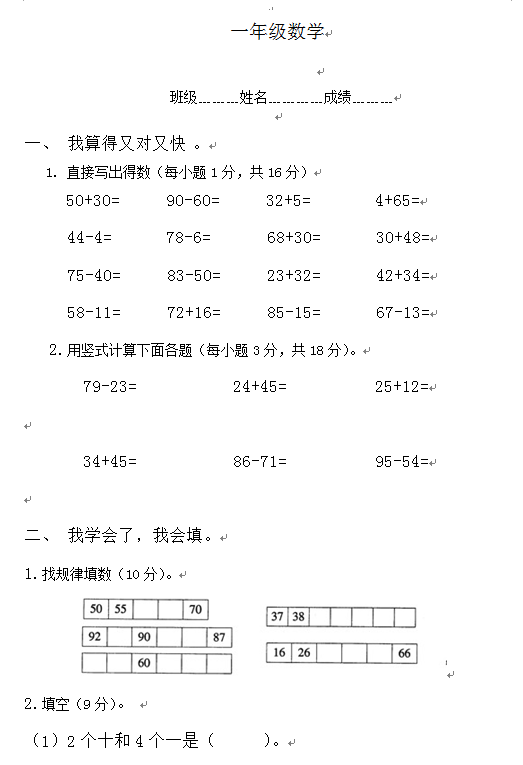1. 直接写出得数（每小题1分，共16分）

50+30=      90-60=      32+5=        4+65=

44-4=       78-6=       68+30=       30+48=

75-40=      83-50=      23+32=       42+34=

【DOC文档28页】小学一年级下册数学期中考试试题A4电子版资料_可直接打印_会员免费下载##### 评论信息